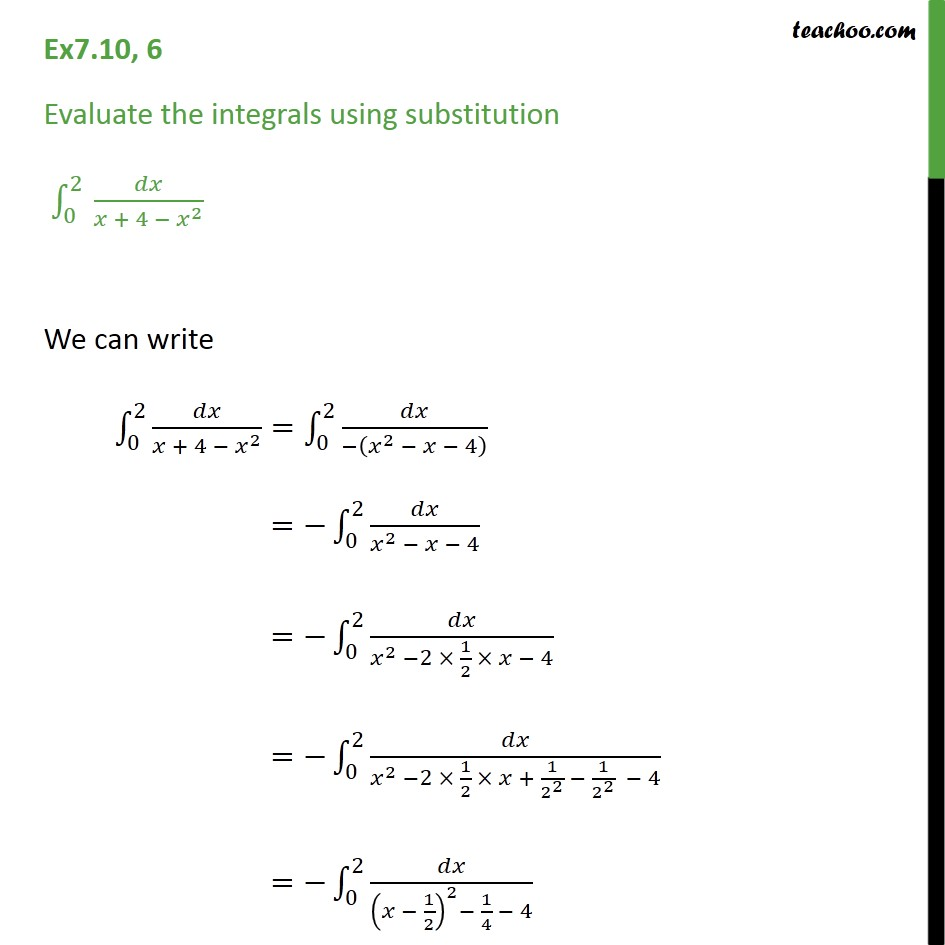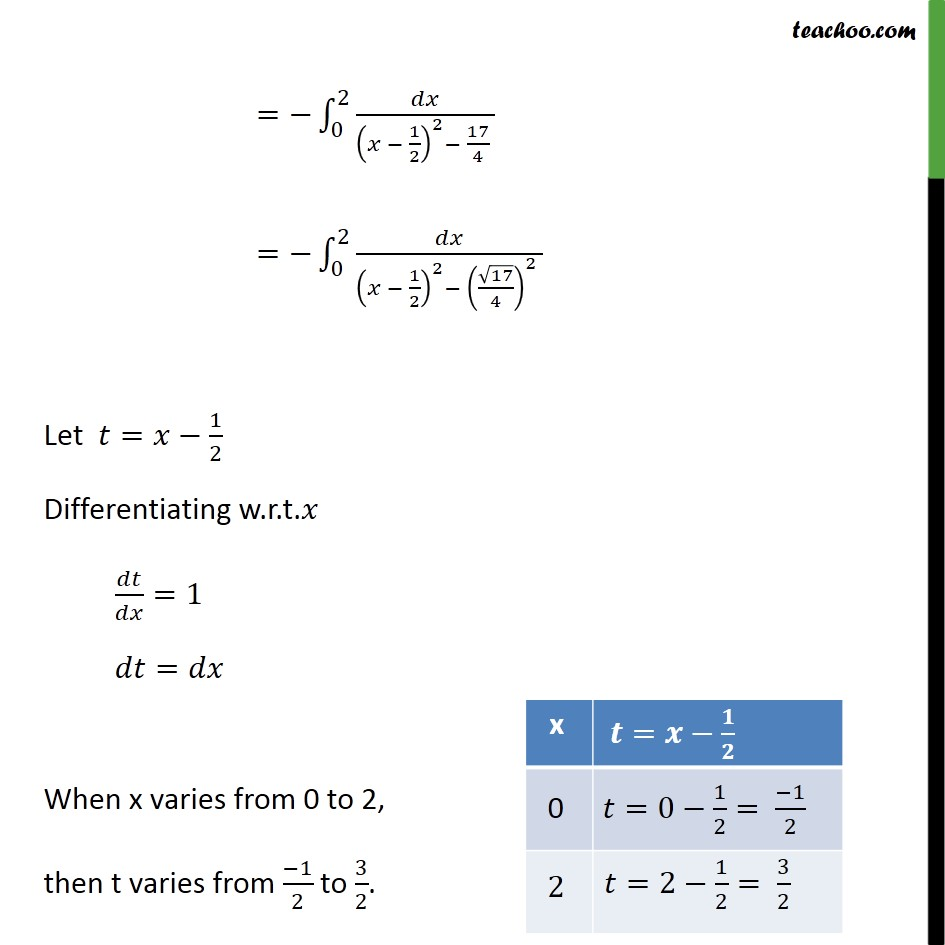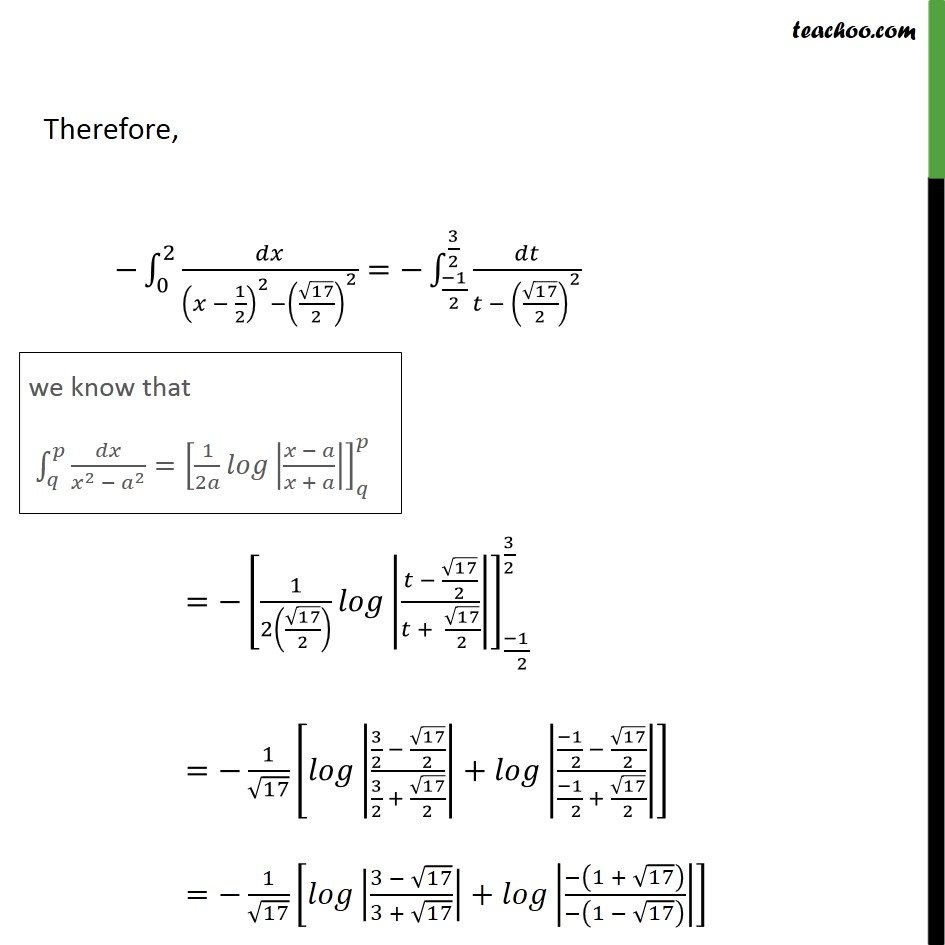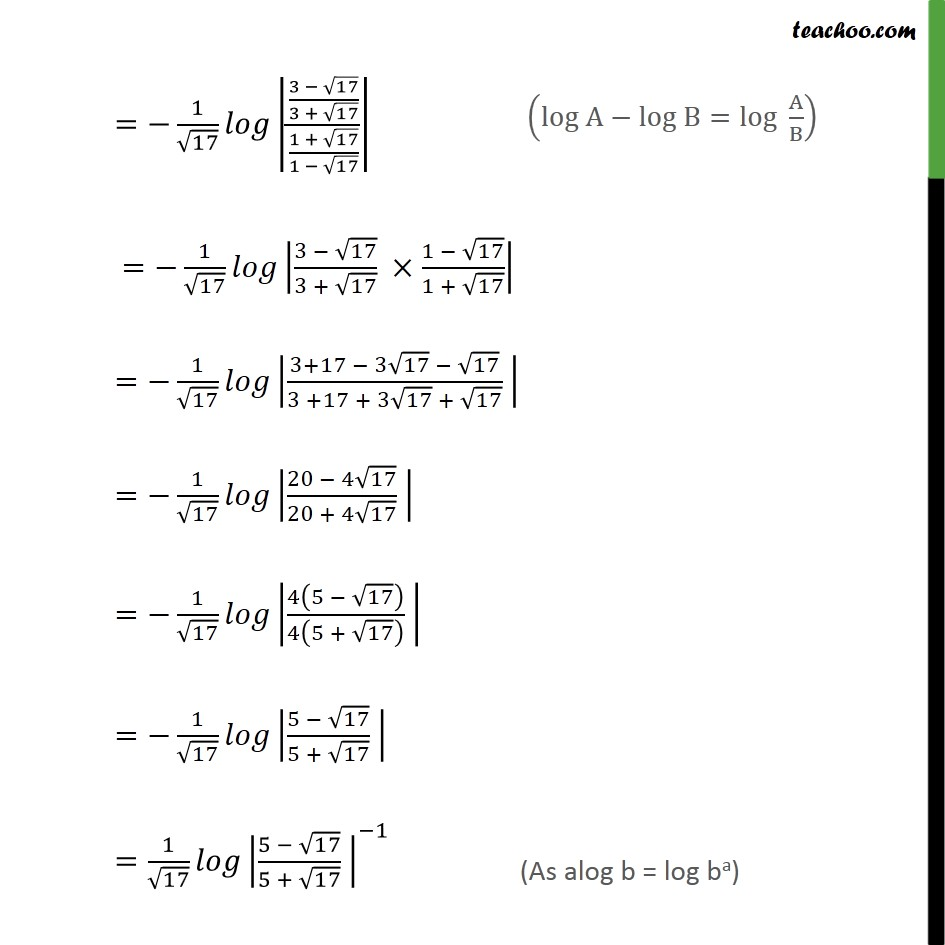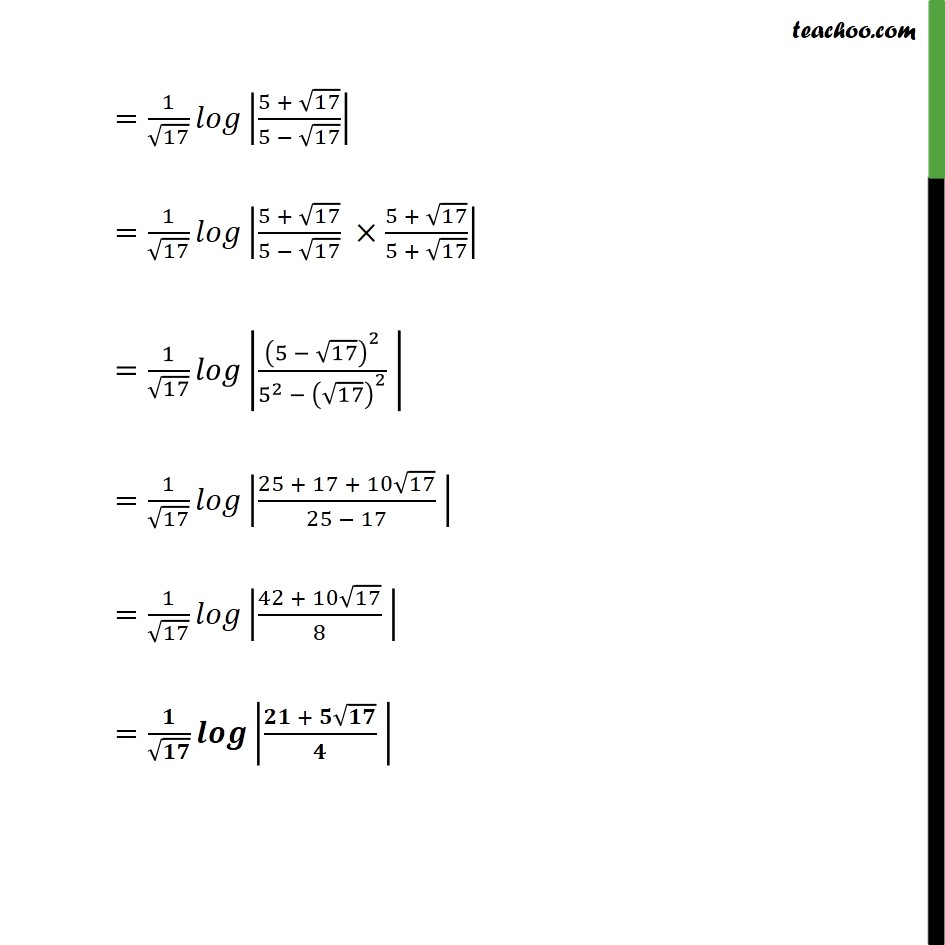1. Chapter 7 Class 12 Integrals (Term 2)
2. Concept wise
3. Definite Integration - By Substitution

Transcript

Ex7.10, 6 Evaluate the integrals using substitution 0 2 + 4 2 We can write 0 2 + 4 2 = 0 2 2 4 = 0 2 2 4 = 0 2 2 2 1 2 4 = 0 2 2 2 1 2 + 1 2 2 1 2 2 4 = 0 2 1 2 2 1 4 4 = 0 2 1 2 2 17 4 = 0 2 1 2 2 17 4 2 Let = 1 2 Differentiating w.r.t. =1 = When x varies from 0 to 2, then t varies from 1 2 to 3 2 . Therefore, 0 2 1 2 2 17 2 2 = 1 2 3 2 17 2 2 = 1 2 17 2 17 2 + 17 2 1 2 3 2 = 1 17 3 2 17 2 3 2 + 17 2 + 1 2 17 2 1 2 + 17 2 = 1 17 3 17 3 + 17 + 1 + 17 1 17 = 1 17 3 17 3 + 17 1 + 17 1 17 = 1 17 3 17 3 + 17 1 17 1 + 17 = 1 17 3+17 3 17 17 3 +17 + 3 17 + 17 = 1 17 20 4 17 20 + 4 17 = 1 17 4 5 17 4 5 + 17 = 1 17 5 17 5 + 17 = 1 17 5 17 5 + 17 1 = 1 17 5 + 17 5 17 = 1 17 5 + 17 5 17 5 + 17 5 + 17 = 1 17 5 17 2 5 2 17 2 = 1 17 25 + 17 + 10 17 25 17 = 1 17 42 + 10 17 8 = +